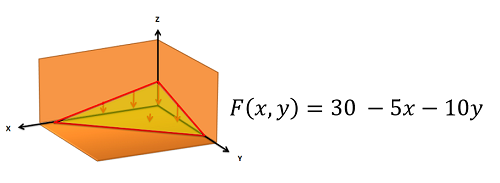﻿ Mechanics Map - Equivalent Point Load via Integration

# Equivalent Point Load (via Integration)

The equivalent point load is a single point force which is statically equivalent to the original distributed force. Being statically equivalent, the equivalent point load will cause the same linear and angular accelerations (on a body that is allowed to move), or it will cause the same reaction forces (if the body is constrained). Finding the equivalent point load for a distributed force often helps simplify the analysis of a system by removing the integrals from the equilibrium equations or equations of motion in later analysis.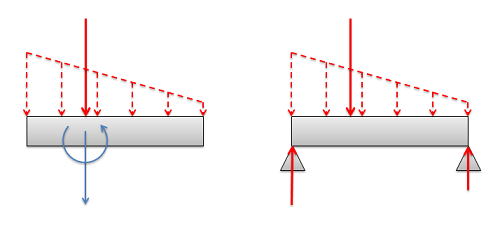If the body is unconstrained as shown on the left, the equivalent point load (shown as a solid vector) will cause the same linear and angular acceleration as the original distributed load (shown with dashed vectors). If the body is constrained as on shown on the right, the equivalent point load (shown as a solid vector) will cause the same reaction forces as the original distributed force (shown with dashed vectors).

## Finding the Equivalent Point Load

When finding the equivalent point load we need to find the magnitude, direction, and point of application of a single force that is equivalent to the distributed force we are given. In this resource we will only deal with distributed forces with a uniform direction, in which case the direction of the equivalent point load will simply match the uniform direction of the distributed force. This leaves the magnitude and the point of application to be found. There are two options available to find these values:

1. We can find the magnitude and the point of application of the equivalent point load via integration of the force functions.
2. We can use the area/volume and the centroid of the area or volume under the force function.

The first method is more flexible, allowing us to find the equivalent point load for any force function that we can make a mathematical formula for (assuming we have the skill in calculus to integrate that function). The second method is usually faster, assuming that we have the available tables of values for areas/volumes and centroids. On this page we will focus on the integration method.

### Using Integration for Distributed Forces in Beams: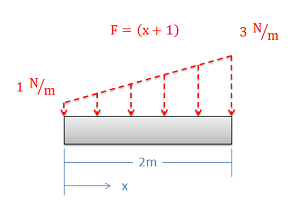The beam shown above has a distributed force acting on it. The force function relates the magnitude of the force to the x position along the top of the box.

We will start by examining a distributed force acting along a single dimension (often represented by a distributed force acting on a horizontal beam). Finding the equivalent point load via integration always begins by determining the mathematical formula that is the force function. The force function mathematically relates the magnitude of the force (F) to the position (x). In this case the force is acting along a single line, so the position can be entirely determined by knowing the x coordinate, but in later problems we may also need to relate the magnitude of the force to the y and z coordinates. In our example, we can relate magnitude of the force to the position by stating that the magnitude of the force at any point in Newtons per meter is equal to the x position in meters plus one. In other words...

 $F(x)=x+1$

Determining the force function is always the first step in finding the equivalent point load via integration, and it is important to get this equation correct. If you start with the wrong equation for the force function, your integration in later steps will not give you valid results.

After finding the force function, the next step is to find the magnitude of the equivalent point load (Feq). The magnitude of the equivalent point load will be equal to the total area under the force function. This will be the integral of the force function over it's entire length (in this case from x = 0 to x = 2).

 $F_{eq}=\int_{xmin}^{xmax}F\left ( x \right )dx$

Now that we have the magnitude of the equivalent point load, we next need to find the location of the equivalent point load. To do this we need to adjust the position (xeq) such that it would cause the same moment as the original distributed force. The moment of the distributed force will be the integral of the force function (F(x)) times the moment arm about the origin (x). The moment of the equivalent point load will be equal to the magnitude of the equivalent point load that we just found times the moment arm for the equivalent point load (xeq). If we set these two things equal to one another and then solve for the position of the equivalent point load (xeq) we are left with the following equation.

 $x_{eq}=\frac{\int_{xmin}^{xmax}\left ( F\left ( x \right ) *x\right )dx}{F_{eq}}$

Finally, with the magnitude, direction, and position of the equivalent point load, we can draw the point load in our original diagram and use this point force in place of the distributed force for further analysis. The equivalent point load can be used for any situation except when examining internal forces (more on that on the internal forces chapter).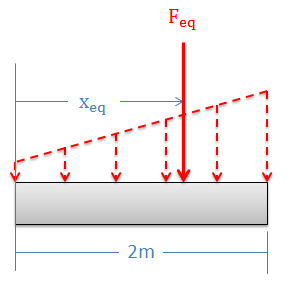The values for Feq and xeq that we have solved for are the magnitude and position of the equivalent point load.

### Using Integration for Discontinuous Force Functions:

Occasionally we will come across a force function (F(x)) that cannot be written out as a single continuous function. In cases like this we will split the function into sections, integrate each section in its given range, and add together the results.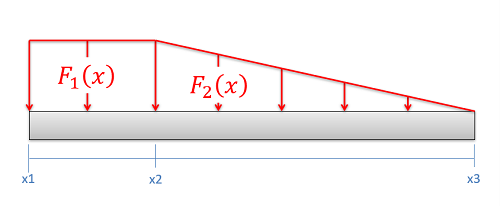Sometimes a single mathematical equation cannot be used to describe the entire function. In cases such as this, it may be necessary to break the function into sections.

Other than doubling all of the integrals, the equations needed to calculate the magnitude and the point of application of the equivalent point load remain more or less the same.

 $F_{eq}=\int_{x1}^{x2}F_{1}\left ( x \right )dx+\int_{x2}^{x3}F_{2}\left ( x \right )dx$ $x_{eq}=\frac{\int_{x1}^{x2}(F_{1}\left ( x \right )*x) dx+\int_{x2}^{x3}(F_{2}\left ( x \right )*x) dx}{F_{eq}}$

### Using Integration for Surface Force Problems: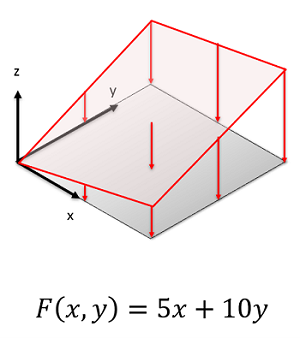The magnitude of the distributed force in this example varies with both x and y coordinates.

With surface force, the force is distributed over a surface, rather than along a single line. To find the magnitude of the equivalent point load we will again start by finding the mathematical equation for the force function. Because the force is distributed over an area rather than just a line, the magnitude of the force may be related to both the x and the y coordinate, rather than just the x coordinate as before.

The magnitude of the equivalent point load (Feq) will be equal to the volume under the force function. To calculate this value we will need to calculate the surface integral, integrating the force function over the entire surface. To do this in practice, we will simply integrate the function F(x,y) twice, integrating with respect to one dimension at a time.

 $F_{eq}=\int\int_{S} F\left ( x,y \right )dA=\int_{ymin}^{ymax}\left ( \int_{xmin}^{xmax} F\left ( x,y \right )dx\right )dy$

Once we solve for the magnitude of the equivalent point load, we can then solve position of the equivalent point load. Since the force is spread over a surface, we will need to calculate both the x (xeq) and the y (yeq) coordinates for the position. To do this, we simply multiply the force function by x or y, then perform a surface integral just as we did in the previous step. Finally, after solving for the surface integral, we divide by the magnitude of the equivalent point load to find the position of the equivalent point load.

 $x_{eq}=\frac{\int\int_{S} \left ( F\left ( x,y \right ) *x\right )}{F_{eq}}$ $y_{eq}=\frac{\int\int_{S} \left ( F\left ( x,y \right ) *y\right )}{F_{eq}}$

## Worked Problems:

### Question 1:

Determine the magnitude and the point of application for the equivalent point load of the distributed force shown below.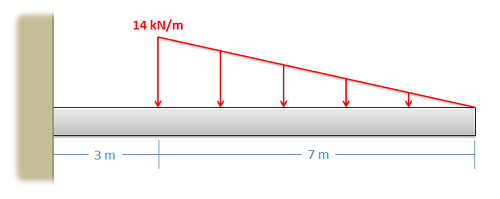### Question 2:

Determine the magnitude and the point of application for the equivalent point load of the distributed force shown below.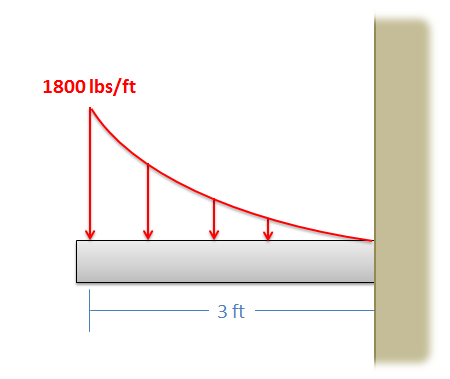### Question 3:

An empty truck trailer with a weight of 17000 lbs is subjected to the wind force shown below. Assuming the weight acts in the middle of the trailer, what is the expected normal forces at the tires on the left and the tires on the right?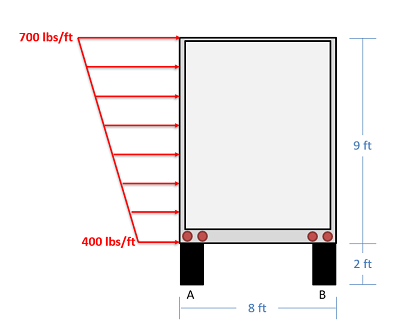### Question 4:

Determine the magnitude and the point of application for the equivalent point load of the distributed force shown below.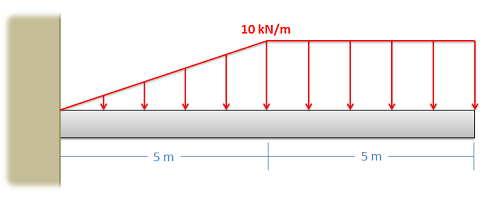### Question 5:

Determine the magnitude and location of the equivalent point load, then use that to find the magnitude of the reaction forces at A and B.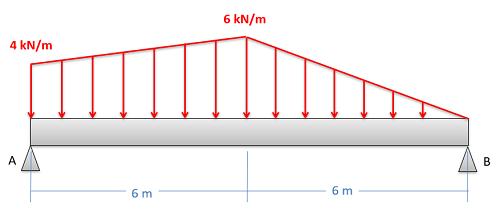### Question 6:

A surface pressure is acting over a 5-meter square area. The magnitude of the pressure, in kPa, is described by the function below. Find the overall magnitude of the force exerted and the location of the equivalent point load.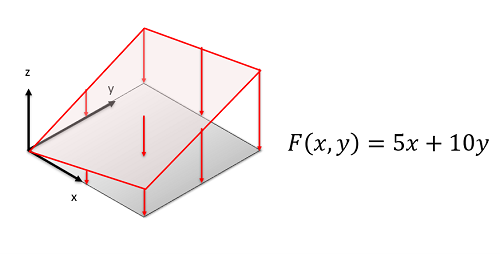### Question 7:

The wind has piled up sand into a corner on a building. The building supervisor is worried about the weight of the sand pushing against the roof of the basement below. The function describing pressure of the sand pushing down on the surface (in pounds per square foot) is given below. Find the magnitude, direction and point of application of the equivalent point load.